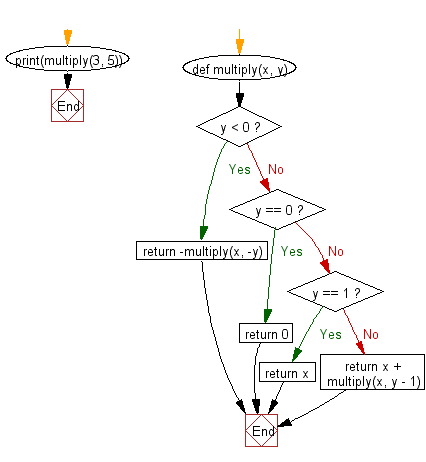﻿ Python Math: Multiply two integers without using the * operator in python - w3resource# Python Math: Multiply two integers without using the * operator in python

## Python Math: Exercise-19 with Solution

Write a Python program to multiply two integers without using the * operator in python.

Sample Solution:-

Python Code:

``````def multiply(x, y):
if y < 0:
return -multiply(x, -y)
elif y == 0:
return 0
elif y == 1:
return x
else:
return x + multiply(x, y - 1)

print(multiply(3, 5));
```
```

Sample Output:

```15
```

Flowchart:## Visualize Python code execution:

The following tool visualize what the computer is doing step-by-step as it executes the said program:

Python Code Editor:

Have another way to solve this solution? Contribute your code (and comments) through Disqus.

What is the difficulty level of this exercise?

Test your Programming skills with w3resource's quiz.

﻿

```>>> students = [{'name': 'John', 'score': 98}, {'name': 'Mike', 'score': 94}, {'name': 'Jennifer', 'score': 99}]